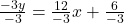## What is the slope of the line -3 y = 12 x + 6?

Question

What is the slope of the line -3 y = 12 x + 6?

in progress 0
2 months 2021-07-25T03:07:02+00:00 1 Answers 4 views 0

Slope = – 4

Step-by-step explanation:

-3y = 12x + 6

Divide the equation by (-3)y = -4x – 2

Compare with y = mx + b

m = -4